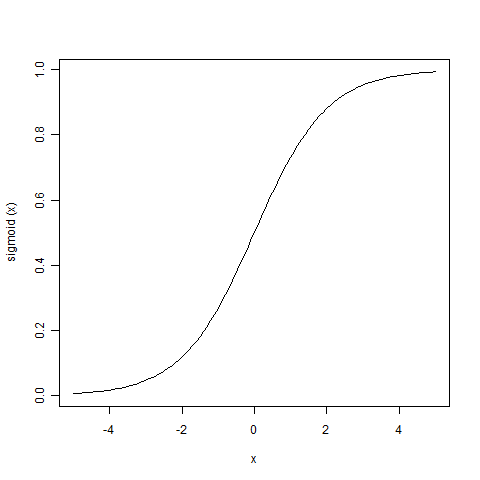# How to Chart a Function in R

R works really well as a quick scientific calculator. I often leave R open simply for quick calculations. R can also be used to quickly graph a function. In this article I will show you how to graph a function with R. The function we will examine is the Sigmoid (or Logistic) function. This function is often used as an activation function for a neural network.

We use the following code to define the sigmoid function.

Once the sigmoid function has been created it can easily be queried as follows.

The above code would produce 0.5.

## Plot a Function

The above function could easily be plotted with the following command.

The -5 and 5 specify the range to plot over. This produces the following plot.

!{Sigmoid function graphed in R}(/images/blog/)

If you would like to save your plot to a file, use the following. The plot will be saved to your documents directory.

The above code was used to produce the plot that you see here.If you would like to plot two equations on the same graph, the following code can be used.